Reach Us+44-1904-929220
On Axionic Dark Matter, Gravitonic Dark Energy, and Multiverse Cosmology in the Light of Non-Linear Electrodynamics | OMICS International
Journal of Astrophysics & Aerospace Technology
All submissions of the EM system will be redirected to Online Manuscript Submission System. Authors are requested to submit articles directly to Online Manuscript Submission System of respective journal.

# On Axionic Dark Matter, Gravitonic Dark Energy, and Multiverse Cosmology in the Light of Non-Linear Electrodynamics

Andrew Walcott Beckwith1 and Lukasz Andrzej Glinka2*

1College of Physics, Chongqing University, Huxi Campus, No. 55 Daxuechen Nanlu, Shapingba District, Chongqing 401331, People’s Republic of China

2Independent Non-fiction Writer and Science Author, Poland

*Corresponding Author:
Editorial Board Member at Journal of Astrophysics and Aerospace Technology
Independent Non-fiction Writer and Science Author, Poland
E-mail: [email protected]

Received Date: December 14, 2014; Accepted Date: April 22, 2015; Published Date: May 20, 2015

Citation: Beckwith AW, Glinka LA (2015) On Axionic Dark Matter, Gravitonic Dark Energy, and Multiverse Cosmology in the Light of Non-Linear Electrodynamics. J Astrophys Aerospace Technol 3: 112. doi:10.4172/2329-6542.1000112

Copyright: © 2015 Beckwith AW, et al. This is an open-access article distributed under the terms of the Creative Commons Attribution License, which permits unrestricted use, distribution, and reproduction in any medium, provided the original author and source are credited.

Visit for more related articles at Journal of Astrophysics & Aerospace Technology

#### Abstract

The non-linear electrodynamics-based approach to a minimum time length, and the axionic Dark Matter (DM) and the gravitonic Dark Energy (DE) are presented in this paper. We approach the DM contribution to the entropy of Universe throughout the Ng quantum infinite statistics, while to obtain a comparative study between entropy in galaxies and entropy of the Universe we analyse the DE in the light of Mishra's quantum formulation of the Big Bang. In result, we receive a non-trivial approach to the initial singularities in the Early Universe cosmology.

#### Keywords

Axion; Big bang; Black hole; Casimir effect; Dark energy; Dark matter; Early Universe; Graviton mass; Infinite quantum statistics; Mach principle; Multiverse hypothesis; Non-linear electrodynamics; Penrose model; Spinning star; Quintessence; Vacuum energy

#### Introduction

In 1972, Zeldovich proposed the methodology of the electronpositron pair production  which can be used to approach the Early Universe cosmology. In this paper, we first discuss this approach in relation to an upper bound of a minimum time length, which compared to certain recent graviton mass study,  creates a productive cosmological scenario. Namely, a non-zero graviton mass gives a non-trivial a minimum scale factor and for a temperature varying cosmological “constant” parameter leads to Quintessence . Afterwards, we first consider more representations of density, and then compare density in the case of certain strengths for the magnetic nonrotating universe. In other words, we involve the weak energy condition versus a more generalized point of view. Furthermore, we examine what the Lagrangian approach gives to the analysis. In the Section1, we analyse how to reconcile a non-linear dynamics with the gravitational physics , whereas, in the Section 2, we study the axionic DM , for which the minimum magnetic field will be crucial, next to our venture of setting up the DM. For this reason, the resulting DM is consistently described throughout axions, with a certain generative entropy. The answer for entropy and a particle mass, is approached , in the matter of a quantum Big Bang, and, moreover, a Machian universe model is applied. Mean while the  and  pertain to a numerical count of entropy, in the Section 2 we study an entropy contribution from both DM and DE. In the Section 3, we show a linkage of this entropy to a ‘particle count’, agreed with the infinite quantum statistics , and the resulting entropy upper bound [7-10], which relates the number of particles to a cosmological constant, and, moreover, directly links DM to an ‘averaged’ particle mass which in turn could lead to many possible values of radius of the universe, that is a specific multiverse model. In the Section 4, we discuss how the DM entropy could inform about the radii of the universe, how compare entropy in galaxies to the Universe entropy, and how to link both DM and DE with the black hole physics, and we briefly study the multiverse scenario.

Minimum time step argument

If we consider the role of an electromagnetic charge, then the derivation due to Zeldovich , including both charged and anti-charged particles, and in an applied electric (E) and magnetic (M) field could yield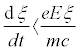(1)

where ξ is an energy expression. To generalize this condition, we consider if E is such that the commensurate bulk charge will be related to the given electromagnetic charge as follows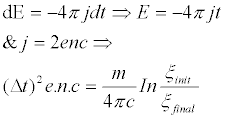(2)

Where n is the number of charges, and if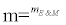is the mass of a hypothetical charge, then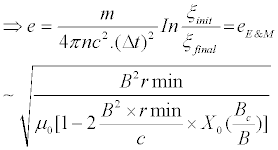(3)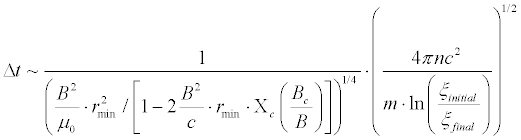(4)

How does the minimum time step (4) relate to absence of the initial singularities of Early Universe? The basic work makes use of the massive graviton mass, , of the following formulation. For a one loop effective action on the Schwarzschild background, the cosmological constant in presence of massive gravitons is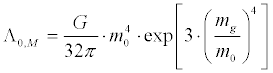(4a)

and aside from the results in the Equation (1), one can compare what is inferred as to its relationship with density with the following directly proportional to an initial density which is directly proportional to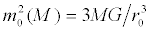(4b)

In the , one can find an expression as to density, with a B field, and we also can use Weinberg's result , of scaling density with one over the fourth power of a scale factor, as well the result of the  for density of a star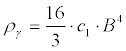(4c)

What we are asserting is, that the very process of an existent E and M field which contributes to a massive graviton in addition to being a Lorentz violation, also, accordingly non-zero initial radii of the Universe. In other words, there are a scaled parameter λ and a parameter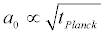paired with α0 which, for the sake of argument, we will set the, with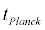~ 10-44 seconds. Also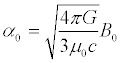(5)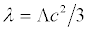(6)

Then if, initially, the parameter (6) is large due to a very large Λ , , that is if the Equation (4) holds, one has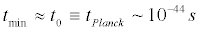(7)

Whenever one sees the coefficient like the magnetic field, with a small initial value, for large values of Λ , this should be the initial coefficient at the beginning of space-time which helps us make sense of the non-zero minimum scale factor,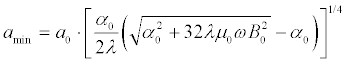(8)

The minimum time, as referenced in the Equation (7) most likely means that the Equation (8) is of the order of about 10-55 , that is 33 orders of magnitude smaller than the square root of the Planck time tPlanck, in magnitude. We next will be justifying the relative size of the large Λ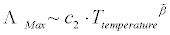(9)

Cf. the Ref. , and we also shall consider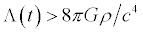(10)

Remarkably, looking at the Equation (9) and the Equation (10), we can see what happens if we look at the Hubble parameter at the start of inflation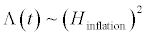(11)

The Equation (9)-(11) argue in favour of a very small scale factor, implying a large density and, moreover, the left hand side of the Equation (1) uses the Equation (9)-(11) regardless of presence the Universe rotation. After that, we should consider what we would do if there is no negative pressure, which leads to a strange situation given by the Equation (2). In that case, with no negative pressure, we get a ‘simple’ temperature dependent massive graviton. We will be examining the import of the Equation (8) from first principles. Note, whether we wish to look at the Equation (2) with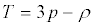(12)

and whether we write a minimum value of the density linked to the cosmological constant in the absence of the magnetic field. In our point of view, this is plausible for zero pressure, which looks strange since in the Early Universe pressure is negative. Moreover, then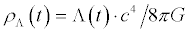(13)

For a non-negative pressure, say zero, one may be able to write, say something not dependent upon the B field, that temperature is dependent on, ,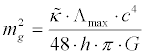(14)

We have reason to believe, though, that this is false, that is that the pressure is negative. Hence, at a minimum, the value of density has a magnetic field component, and in the Equation (1) the relevant density may be the one obtained by reciprocal of the fourth power the Equation (8), due to the Equation (5). If we do so, then possibly we are assuming that there is no rotation of the universe.

If there is a rotation of the universe, we may up to a point treat the density as what was done in the  for stars, that is examine if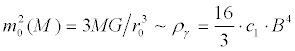(15)

For a non-rotating universe, one has, Cf. the Ref. ,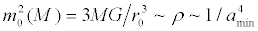(16)

The minimum scale factor in the Equation (16) has a complicated magnetic field dependence as given in the Equation (8). In the , there is a generalized density case. This is valid if we have a nonrotating universe, and otherwise, we should use the results of the  for density. Notably, for absence of rotation, the density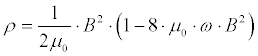(17)

has a positive value only if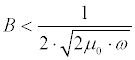(18)

In our opinion, the Equation (16) is more general, although the magnetic field dependence is far more complicated. The question is to find a relevant Lagrangian, used for analyse of both the minimum radius problem and the gravitation in the cosmological model. First of all, for a spinning star, [15,16], we believe that the complementary are the [17,18]. Ultimately, the material in the  as to a Goldstone theorem and non-linear E and M analysis can be crucial. Namely, for using the formalism of the , we need to determine if the universe is a spinning star. If not, then the density to consider is in Equation (1), not the cosmological constant, is like the value given in Equation (16), and relates a massive graviton to the cosmological constant. Otherwise, , density is determined as in the Equation (15) Then, the Lagrangian is given as in the [17,18] with all issues addressed, as far as an appropriate Goldstone-like theorem, and its connections to a non-linear electrodynamics. In fact, for a rotating universe, a bridge between gravity and non-linear electrodynamics, and energy loss due to quantum vacuum friction which can be present in graviton production, exists. Note that [17,18], a generalized Lagrangian for a rotating star is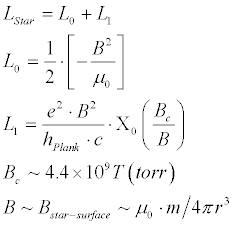(19)

where the function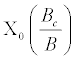is defined in the Ref. , and in presence of quantum vacuum friction, then the decay in rotation of the star is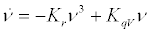(20)

with the coefficients in the right hand side defined in the [17,18].

Our supposition is that, for a rotating universe, one has a magnetic field generated and a set of the Lagrangian, partially similar to the Equation (19), and that the Equation (20) would hold but, of course, we would need a very different moment of inertia for a non-zero radius, but still rapidly spinning star-like Early Universe, space-time which would be still decaying, according to the Equation (20). In our opinion, quantum vacuum friction for a rotating universe may lead generate gravitons according to the Goldstone theorem developments . If for a rotating universe there is a decay similar to the Equation (20), then we may see a natural solution to power loss which will form the Goldstone modes, [17,18], and, in the case of a Lorentzian fourdimensional space-time, only two inequivalent vacua exist. Moreover, if a graviton mass should be constrained experimentally on the order of 10-62 g, then one can determine either quintessence or lack of time varying cosmological constant experimentally.

We submit that this inquiry and a choice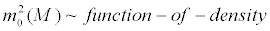, as in the Equation (1), will lead to falsifiable experimental conditions on determination of both Quintessence as well as presence of rotation. If rotation is absent, then this will be very helpful to obtain experimental verification of a graviton mass. Otherwise, we turn back to the results of the . For this reason, this detail should be settled as soon as possible, and is particularly important in experimental verification of the theoretical cosmological sense of a non-linear electrodynamics. Note that, if every spinning star in itself generates an Early Universe, that is the Equation (4) is intuitively useful, then the negative pressure requirement is needed to start the inflationary epoch, and, otherwise, the pressure not only is strongly signed throughout the non-linear electrodynamics, but also requires, especially within the Planck regime of space-time, a much more complicated behaviour of a magnetic field and, for this reason, more complex empirical ideas.

Axionic dark matter

In the , one million or more black holes in the centre of an equal number of galaxies lead to an entropy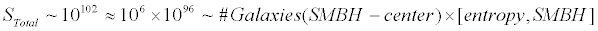(21)

To understand what this means, we will review our version of the quantum Big Bang , in order to coin a self-consistent axionic DM. Applying the Mach principle, with M being the mass of the spherical universe of a radius 0 R , one has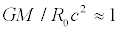(22)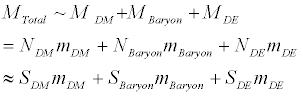(23)

where the total entropy of the Universe is assumed to be in the presentday era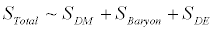(24)

The use of entropy as akin to particle count comes from two sources. First scenario is due to ‘infinite quantum statistics’, , that is begin with a partition function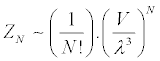(25)

and leads to entropy of the limiting value of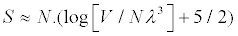(26)

Because of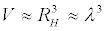, so unless N close to 1 contradicts a negative entropy, and, for this reason, the N! term is removed to receive a quantum Boltzmann statistics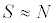(27)

Alternatively, in the scenario of late time cosmological constant behaviour, one has, ,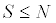(28)

and, moreover, ,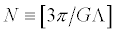(29)

refers to total number of degrees of freedom which will be shown to become enormous for sufficiently small Λ , Cf. the . Pending a review of the situation, the following could be entertained [19,20]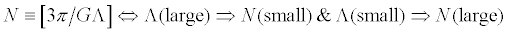(30)

Specifically, this refers to DM, and helps to answer our question if DM is the preferred venue for explaining the behaviour of the Equation (30). In fact, if there is Quintessence as given by the Equation (9), then this may be able to explain why there is

Λ(field− theory) ~ 10122 ×Λ(actual − today) (31)

There is a linkage of black hole entropy with Λ as vacuum energy [20,21], and L as a spatial length associated with black holes L3Λ3 ≤SBH=πL2M2p, L3Λ4 ≤ LM2p, Smax ~ SBH3/4. Furthermore, Figure 1 indicates that DE can be created in a later cosmological evolution rather than in the Early Universe. If DE is non-gravitonic, then either Quintessence, as is indicated here, or else one of the Chaplygin gas models of DMDM, with DM and DE as different facets of the same evolutionary cosmological dynamic, should be considered. The gravitonic DE could determine applicability of the multiverse scenario. The multiverse hypothesis could give a physical sense to a graviton mass, and enlighten how the quantum cosmological scenario , works in dependence on the entropic algorithm. We argue that if there is temperature dependence as in the Equation (9) and if N bounds entropy and is as in the Equation (29), then according to the Figure 1, DM is not affected while vacuum energy gives DE.

What we see in the aforementioned arguments, is that N as given by the Equation (29), with Λ given by the Equation (9), with the Equation (11) as backup is an artefact of DE, not DM, and that as a result, the Figure 1 shows that time-dependent N is due to Quintessence at least in the Early Universe. As of 13.7 billion years ago, the background temperature given by first light about 380 thousand years after the Big Bang was 105Kelvin , [21,22], as opposed to the present-day 3 Kelvins of the cosmic background radiation, so if the Equation (9) is applied, then, if we associate Λ with DE, one has ΛDE(105 Kelvin) << ΛDE(3 Kelvin) and, therefore, NDE(105 Kelvin) >> NDE(105 Kelvin). The hypothesis of the Equation (9) complemented by the Figure 1, argues strongly against vacuum energy-generated DM. Concerning the 380 thousand years after the Big Bang, one has MTotal ~ MDM + MBaryon + MDE ~ MDM + MBaryon ~ NDM mDM+ NBaryonmBaryon ~ SDM mDM+ SBaryonmBaryon. With R as the presumed present radius of the Universe, and m is the mass of an ‘average’ constituent particle, and N is the number of particles in the universe, with tau being the time after the Big Bang to the present era, one has the values given in Table 1.

m×10−35 g R×1028cm N ×1091 Mtotalx1056pm ×10 yr
1.07299 1.896 2.38429 2.5582 20
1.23891 1.422 1.54865 1.91875 15
1.51744 0.948 0.84297 1.27916 10
2.14598 0.474 0.29804 0.639588 5

Table 1: From S. Mistra .

If the Figure 1 scenario is valid 380 thousand years after the Big Bang, then a total mass of the universe M does not change and, , the average particle of the quantum universe is of the order of magnitude of axionic DM particle, that is 1.23×10-35 g ~ 5.609×10-2eV , while the entropy is counted by ‘averaged’ number of particles, axionic energy has a range of 10-6-20 eV, and by the Figure 1 and Table 1, SDM ∝1090 −1091 . For axionic DM, by Figure 1, DE is roughly 3-4 times more mass than DM. The  brings this idea throughout the black hole atoms known as the maximons or friedmons, the particle-like gravitating objects (semi-closed worlds) of mass close to the Planck mass, which may have a large gravitational mass defect and may create DM. Notably, microblack holes carrying the electric charge and having the orbiting either electrons or protons outside the horizon orbits are the basis of the Hawking radiation. For this scenario, we should consider the feasibility of both the formation and destruction of most cosmological matterenergy by black holes. Moreover, just black holes, as intense generators of gravitational waves, may be linked to gravitons in general.

Gravitonic dark energy

Let us consider gravitonic DE, [22,23]. If a graviton mass is 2·10-62 g ~ 2.8· 10-30 eV, then in the present-day era SDE ~ 10117-10118. In other words, if the Eqs. (29)-(31) with an initial temperature of 3 Kelvin are valid, then SDE ~ 1090-1091 would hold. If one applies the temperature value 1032 Kelvin in the Eqs. (29)-(31), then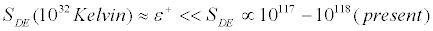. For massless gravitons, Mishra's analysis does not apply to DE and gravitonic DE is nonphysical, while becomes potentially physical for a graviton mass 2·10-62 g ~ 2.8· 10-30 eV. Then, one can look for another DE, for example if the DE density is constant, that is the curvature of space-time is constant, which means that the universe expands at a fixed rate Figure 2.

The question is DE density for gravitonic DE. The  uses the Casimir effect-generated DE, where massive gravitons are fermions rather than bosons, and, therefore, a particle DE idea is unnecessary and Mishra's idea for DE collapses. However, , regardless of a graviton mass, and even physical nature of gravitons in general, if it is the observable DE, then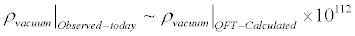and the factor of 10112 for difference in energy density due to the vacuum energy should be compared with DE entropy calculated with the present-day DE entropy value SDE ~ 10117-10118 created by a graviton. The value of 10112 versus 10118 is a problem fr discussion.

Super massive black holes versus the Universe

The Penrose multiverse scenario

We can assume that there are possibly many more than one million black hole-like galaxies, that is 106 to 1020 super massive black holes in the centre of a galaxy, and that up to a point, the entropy of a super massive black hole in the centre of a galaxy is at most 10112. According to the , one has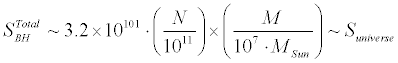and if this is true, then the cosmological sense of M ~ 1010 MSUN → N ~ 1020-1023 is the question. In other words, then the numerical factor is so high that indeed the multiverse hypothesis for entropy can be right with a small graviton mass. The cyclic universe hypothesis, , in itself is a specific realization of the multiverse hypothesis concerning presence of many universes. Throughout black hole evaporation and the embedding structure of our universe, one can easily see that the Penrose model embeds black holes and may resolve the dichotomy that there are no fewer than N universes undergoing the ‘infinite expansion’, [9,27], contained in a mega universe structure. Every universe has a black hole evaporation, and the decaying black holes display the Hawking radiation. If each of the N universes is defined by a partition function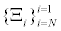then there is an information ensemble of mixed minimum information correlated as about 107-108 bits per partition function in the set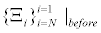, so minimum information is conserved between a set of partition functions per universe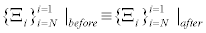. However, the information put into each partition functionis non-unique. Furthermore, the Penrose's Hawking radiation is collated via a strange attractor collection in the mega universe structure to form a new Big Bang for each of the universes. Verification of this mega structure compression and expansion of information with a nonunique information placed in every universe favours ergodic mixing treatments of initial values for every universe expanding from an initial singularity. The nf, with Sentropy ~ nf , as in the [6,26]. How to tie in this energy expression, as in the Equation (30) will be to look at the formation of a non-trivial gravitational measure as a new Big Bang for every universe as by n(Ei), the density of states for a partition function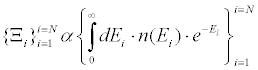where Ei is with the iteration energy for N universes, Cf. the Refs. [9,27], and then,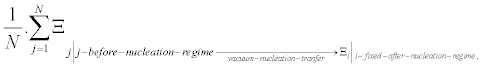where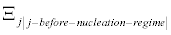is the partition function of a j-th universe, constructed by the absorption of one to ten million black holes taking in energy, prior to the evolution to the present-day Universe. Furthermore, by the ,in terms of general ergodic mixing. The question is a protocol as to how a multidimensional representation of black hole physics enables continual mixing of space-time largely as a way to avoid the anthropic principle, as to a preferred set of initial conditions. This complex multiverse allows bridging what seems to be an unworkable dichotomy between ultra-low graviton frequency, corresponding roughly to a reast mass 10-65 g, easily satisfied by the Kerr black holes with rotational frequencies, as given in out text as many times greater, combined with the absurdity of what is the Equation (29). How can a graviton with a wavelength 10-4 the size of the universe interacts spatially with a Kerr black hole. Embedding the black hole into a multiverse setting may be the only way out. The idea here is to use what is known as CCC cosmology. Namely, having a Big Bang for the universe, after redshift gains the value z=10, a billion years ago, the formation of super massive black holes starts and matter energy is vacuumed up, which at a much later date than in the presentday era gather up all the matter-energy of the Universe and recycles it in a cyclic conformal translation given by the Einstein field equations with the cosmological constant like in the Equation (9). Then the main methodology is evaluation of a change in the metric gab by a conformal mapping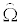to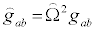. Penrose utilized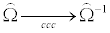, and in fall into cosmic black holes the Penrose hypothesis could be recycled when the caveat that the Planck constant is still a constant from cycle to cycle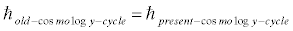, that is throughout generalization by a weighing averaging. Interestingly, changing this cycle would lead to most universes having a non-consistent cosmology, that is not adhering to this rule would not be stable and would collapse and fail to evolve.

#### Conclusion

If there are massive gravitons as well as Mishra’s analysis holds, with a Machian principle saved, then the multiverse is existent. Secondly, a straightforward application of the Mishra approach, for massless gravitons, yields an average mass of the universe consistent with axionic DM, and then the gravitonic DE is physically consistent. DM could be formed by mini black hole atoms, but this suggestion appears to be contravened by the Mishra model which requires the Mach principle. Whether this Machian dynamics for the present universe should not hold is the question, and if the answer is positive then this the Mishra approach is consistent. Also, the implications of ergodic mixing are valuable, and, in our current views, should be further developed, . If the multiverse hypothesis is to be entertained and investigated, seriously, then another older idea should be vetted and explored. As given in the , open universes form bubbles, this would be using the Bunch Davies vacuum fluctuation, and the question would be identification of different bubbles which may have starting points [9,10,27]. Moreover, the question of axionic gravitons have already been discussed [29-32], and this model opens the way to approach DM and DE in the framework of the multiverse hypothesis in the light of quantum field theory, what makes this approach potentially productive for a further discussion.

#### Acknowledgement

A.W. Beckwith thanks the National Nature Science Foundation of China for supporting this work (grant No. 11375279). L.A. Glinka thanks A.W. Beckwith for invitation to co-authoring this work.

#### References

Select your language of interest to view the total content in your interested language

### Article Usage

• Total views: 12870
• [From(publication date):
June-2015 - Sep 16, 2019]
• Breakdown by view type
• HTML page views : 9041

## Post your commentCan't read the image? click here to refresh
###### Peer Reviewed Journals

Make the best use of Scientific Research and information from our 700 + peer reviewed, Open Access Journals

International Conferences 2019-20

Meet Inspiring Speakers and Experts at our 3000+ Global Annual Meetings

Top